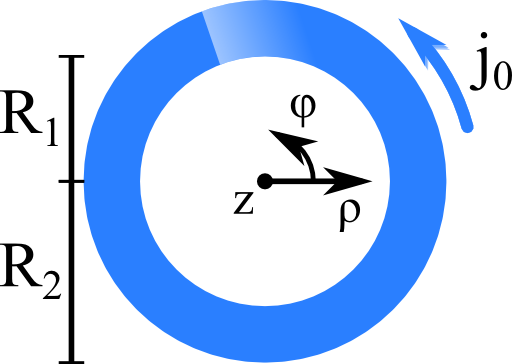## A Cylinder Shell Current and its Magnetic FieldAmpère's law is hard to solve in general. What if certain symmetries of a current distribution are present? We may find much simpler differential equations which might be integrated directly. Find out what to do in rotational and translational symmetry!

## Problem StatementLet us assume a "cylinder shell current distribution" of the following form:$\begin{eqnarray*} \mathbf{j}\left(\mathbf{r}\right)&=&\begin{cases} j_{0}\mathbf{e}_{\varphi} & \rho\in\left[R_{1},R_{2}\right]\\0 & \text{else}\end{cases}\ . \end{eqnarray*}$This current distribution is rotationally symmetric which means that it does not vary in $$\varphi$$. Furthermore, the current distribution is infinitely extended in the $$z$$-direction. This is called a translational symmetry.

Use Ampère's law to determine the magnetic field $$\mathbf{H}\left(\mathbf{r}\right)$$ everywhere for the given current distribution.

If you found a solution for the cylinder shell current distribution, how would you determine the magnetic field of a more general rotationally and translationally invariant current distribution, i.e. $\mathbf{j}\left(\mathbf{r}\right) = j\left(\rho\right)\mathbf{e}_{\varphi}\ ?$